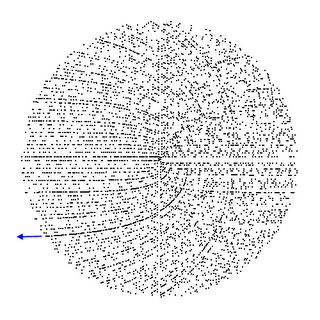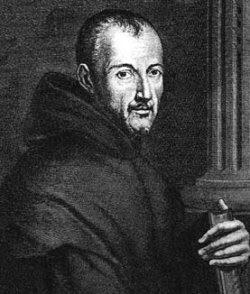## Saturday, January 06, 2007

### Mersenne Prime: One < the Power of twoIt looks as though primes tend to concentrate in certain curves that swoop away to the northwest and southwest, like the curve marked by the blue arrow. (The numbers on that curve are of the form x(x+1) + 41, the famous prime-generating formula discovered by Euler in 1774.)

This is part of the education of my learning to understand the implications of the work of Riemann in context of the Riemann Hypothesis. Part of understanding what this application can do in terms helping us to see what has developed "from abstractions of mathematics," to have us now engaged in the "real world" of computation.

In mathematics, a power of two is any of the nonnegative integer powers of the number two; in other words, two multiplied by itself a certain number of times. Note that one is a power (the zeroth power) of two. Written in binary, a power of two always has the form 10000...0, just like a power of ten in the decimal system.

Because two is the base of the binary system, powers of two are important to computer science. Specifically, two to the power of n is the number of ways the bits in a binary integer of length n can be arranged, and thus numbers that are one less than a power of two denote the upper bounds of integers in binary computers (one less because 0, not 1, is used as the lower bound). As a consequence, numbers of this form show up frequently in computer software. As an example, a video game running on an 8-bit system, might limit the score or the number of items the player can hold to 255 — the result of a byte, which is 8 bits long, being used to store the number, giving a maximum value of 28−1 = 255.

I look forward to the help in terms of learning to understand this "ability of the mind" to envision the dynamical nature of the abstract. To help us develop, "the models of physics" in our thinking. To learn, about what is natural in our world, and the "mathematical patterns" that lie underneath them.

What use the mind's attempt to see mathematics in such models?

"Brane world thinking" that has a basis in Ramanujan modular forms, as a depiction of those brane surface workings? That such a diversion would "force the mind" into other "abstract realms" to ask, "what curvatures could do" in terms of a "negative expressive" state in that abstract world.

Are our minds forced to cope with the "quantum dynamical world of cosmology" while we think about what was plain in Einstein's world of GR, while we witness the large scale "curvature parameters" being demonstrated for us, on such gravitational look to the cosmological scale.

Mersenne PrimeMarin Mersenne, 1588 - 1648

In mathematics, a Mersenne number is a number that is one less than a power of two.

Mn = 2n − 1.
A Mersenne prime is a Mersenne number that is a prime number. It is necessary for n to be prime for 2n − 1 to be prime, but the converse is not true. Many mathematicians prefer the definition that n has to be a prime number.

For example, 31 = 25 − 1, and 5 is a prime number, so 31 is a Mersenne number; and 31 is also a Mersenne prime because it is a prime number. But the Mersenne number 2047 = 211 − 1 is not a prime because it is divisible by 89 and 23. And 24 -1 = 15 can be shown to be composite because 4 is not prime.

Throughout modern times, the largest known prime number has very often been a Mersenne prime. Most sources restrict the term Mersenne number to where n is prime, as all Mersenne primes must be of this form as seen below.

Mersenne primes have a close connection to perfect numbers, which are numbers equal to the sum of their proper divisors. Historically, the study of Mersenne primes was motivated by this connection; in the 4th century BC Euclid demonstrated that if M is a Mersenne prime then M(M+1)/2 is a perfect number. In the 18th century, Leonhard Euler proved that all even perfect numbers have this form. No odd perfect numbers are known, and it is suspected that none exist (any that do have to belong to a significant number of special forms).

It is currently unknown whether there is an infinite number of Mersenne primes.

The binary representation of 2n − 1 is n repetitions of the digit 1, making it a base-2 repunit. For example, 25 − 1 = 11111 in binary

So while we have learnt from Ulam's Spiral, that the discussion could lead too a greater comprehension. It is by dialogue, that one can move forward, and that lack of direction seems to hold one's world to limits, not seen and known beyond what's it like apart from the safe and security of home.